Counting Creatures

This section describes what Palago creatures are, how to count them and how many there might be.

1.  What is a Creature?

A creature is any closed shape that can be formed by Palago tiles of matching colour in a hexagonal grid. Tiles may exist in one of three rotations {a, b, c} relative to each other.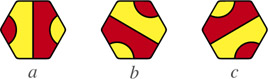Creatures may contain subcreatures (below, left). Creatures may surround holes (below, right) provided that no part of the creature itself opens onto empty space.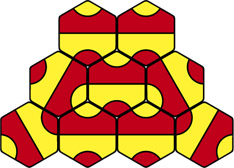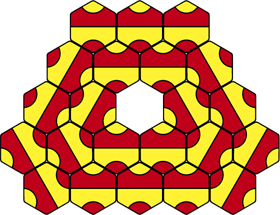Red creature containing a yellow subcreature. Red creature surrounding a hole.

A creature is described as filling its tile set if the removal of any tile will break it. For example, the red creature on the left (above) fills the left tile set but the contained yellow subcreature does not. The outer boundary of a full creature will visit every exterior tile.

2.  Creature Sums

Each creature is identified by a unique algebraic sum. For example, the following two creatures are described by the sums "4b+5c" and "0a+1c+2a+2c+2c+2c+3b+2c3a+4a----3b".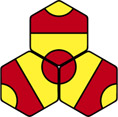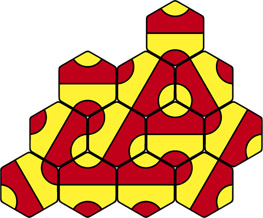Creature: 4b+5c. Creature: 0a+1c+2a+2c+2c+2c+3b+2c3a+4a----3b.

Each sum is a set of instructions for recreating that individual, using the following tokens:

•  {0,1,2,3,4,5}    Turn a number of 60 degree increments (clockwise).
•  {a,b,c}             Add a new tile at the current position.
•   +                   Step into the newly created tile.
•   –                   Step out of the current tile.

For example, the recontruction of creature 4b+5c (the Eye) is shown below. Starting with an initial tile a and a pointer in the default (up) position, each token is processed in turn. The first token '4' rotates the pointer four steps clockwise, the next token 'b' adds a new tile b at the position currently pointed to, the next token '+' steps the pointer into the newly created tile (and points it back to the direction it came from), the next token '5' rotates the pointer five steps clockwise, and the final token 'c' adds a new tile c at the position currently pointed to.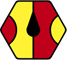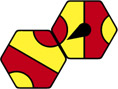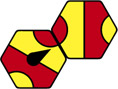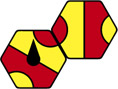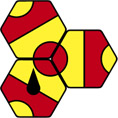Start. '4': rotate four steps. 'b': add new tile b. '+': step into new tile. '5': rotate five steps. 'c': add new tile c.

Creature sums describe full creatures only, and a creature's size equals the minimum number of tiles required to create it. The number of letters and number of characters in each creature's sum will both be exactly one less than its size, due to the assumed initial tile.

Creature sums are invariant to translation and rotation as the instructions are relative to the initial tile at the default rotation. Different sums may be derived for the same creature depending on starting point, so the least-valued of these (using stringwise comparison) is chosen to represent each creature. This representative sum is linked to the sum of that creature's reflection to also ensure reflection invariance.

3.  Counting Creatures

The above method for uniquely labelling creatures makes it possible to enumerate all individuals up to a given size. An efficient way to count creatures is to construct them directly by starting with the simplest creature (the Eye) then iteratively developing more complex shapes from it and its successors.

The following example shows how to develop a creature into a more complex one by: 1) rotating two adjacent exterior tiles, and 2) adding a further tile to close it again. All unique combinations of one or two adjacent exterior tiles in all rotations must be tried in order to guarantee that all possible creatures are created, and only the minimum number of tiles required to close the creature should be added.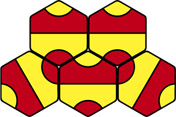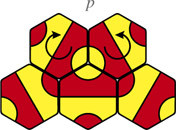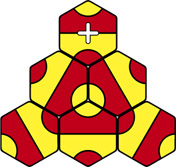A full creature... ...two tiles rotated to open it... ...and one tile added to close it again.

The enumeration method is summarised in pseudocode as follows:

```    add simplest creature (4b+5c) to queue Q
while (Q not empty)    {        get creature c from front of Q
if (c.size < target size)
{
for (all combinations of all rotations of one or two adjacent exterior tiles)
{
open c
close again by adding the minimum number of tiles -> c'
if (c' is full and not in Q)
add c' to end of Q
}
}
} ```

4.  Creature Counts and Estimates

The following table shows the numbers of full creatures to size 20. Only red creatures are counted; there will be an equal number of yellow creatures.

 Size Creatures Found Computation Time Listings h =  1 0 < 0.001s – h =  2 0 < 0.001s – h =  3 1 < 0.001s creatures-size-03.txt h =  4 0 < 0.001s – h =  5 1 < 0.001s creatures-size-05.txt h =  6 1 < 0.001s creatures-size-06.txt h =  7 2 < 0.001s creatures-size-07.txt h =  8 2 0.016s creatures-size-08.txt h =  9 9 0.031s creatures-size-09.txt h = 10 13 0.063s creatures-size-10.txt h = 11 37 0.172s creatures-size-11.txt h = 12 81 0.468s creatures-size-12.txt h = 13 205 1.28s creatures-size-13.txt h = 14 512 3.95s creatures-size-14.txt h = 15 1,335 12.2s creatures-size-15.txt h = 16 3,404 41.0s creatures-size-16.zip h = 17 8,922 2.61m creatures-size-17.zip h = 18 23,592 14.1m creatures-size-18.zip h = 19 62,630 1.39h creatures-size-19.zip h = 20 167,622 9.41h creatures-size-20.zip

It can be seen that the number of creatures slightly more than doubles with the addition of each tile, but that the time required to count them increases more dramatically. The progression of creature numbers is approximated by the equation: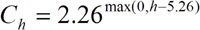where h is the number of tiles and Ch is the estimated number of creatures of that size. This allows the extrapolation (with a grain of salt) of approximate creature counts for larger numbers of tiles that would take too long to count exactly. Of special interest is the number of creatures possible with a complete set of 48 Palago tiles.

 Size Expected Creatures h =  25 9,775,000 h =  30 576,000,000 h =  35 34,000,000,000 h =  40 2,000,000,000,000 h =  48 1,360,000,000,000,000

This suggests that there may be over a quadrillion distinct creatures within each bag of 48 tiles. The total estimated count of all creatures for sizes 1..48 is 2,450,000,000,000,000.

5.  The Zoo

The 29 creatures to size 10 are listed below. Each creature is named for convenient reference.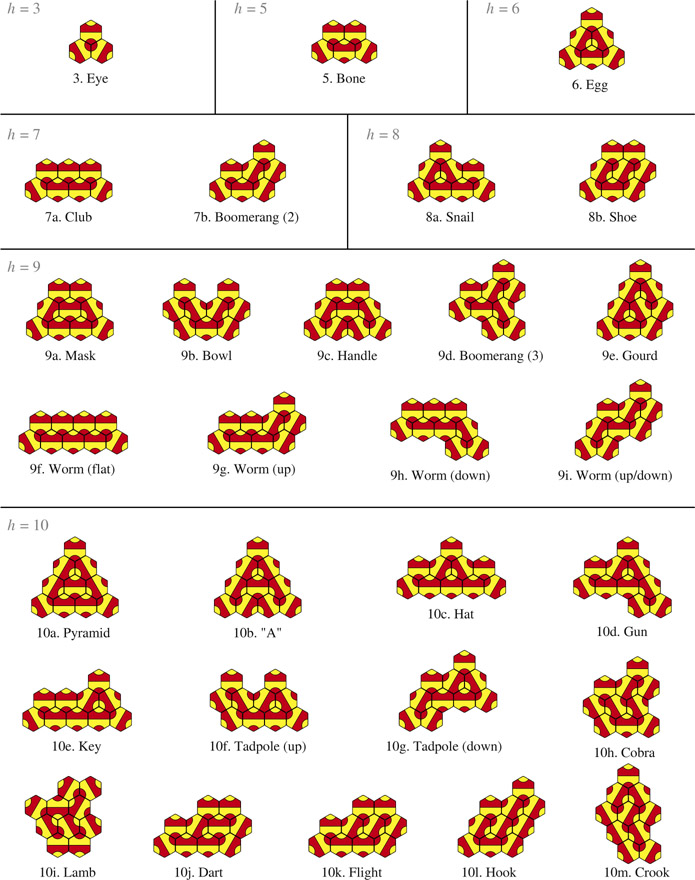6.  Creatures and Polyhexes

There is an obvious relationship between polyhexes (connected sets of hexagons) and creatures. Note, however, that distinct creatures may occupy the same polyhex silhouette – for example 10a/10b or 10j/10k – hence it is not sufficient to distinguish creatures by polyhex description.

Notating the rotation of each external tile is not sufficient either, as this can fail to distinguish key internal topology. For example, the following two tile sets have identical exterior tile rotations but the set on the left contains one full creature while the set on the right contains two non-full creatures, due to the rotated internal tile. The rotation of every tile must therefore be described, as per the above labelling method.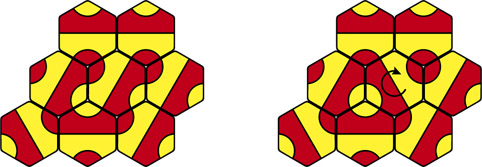Two tile sets with the same outline chain code but of significantly different topology.

7.  SLR Contour Codes

If only the outlines of creatures are required, SLR contour codes are a convenient way to label and distinguish them. SLR stands for {straight, left, right} which are turtle instructions for reconstructing the contour. For example, the following contour consists of the following segments (starting at the arrow): left, left, right, right, straight, right, straight, etc. This may be abbreviated and the entire contour described as: llrrsrsrllsrslrrsssrs.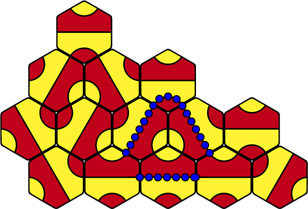Starting point for contour llrrsrsrllsrslrrsssrs. Body segments (dotted).

Only the longest closed contour is labelled for a given creature, and this will always be its outer contour. SLR contours should always be oriented clockwise (more right segments than left segments) and the starting point chosen to give the lowest alphabetical label.

It can be useful to know which segments of a contour describe the creature's body and which its limbs. Body segments (shown dotted above, right) are indicated by capitalisation: llrrsrsrllSRSlrrsSsrs. The following method distinguishes body and limb segments of a given SLR contour:

```    make all segments uppercase
for each consecutive {R, R} pair    // limb extremity    {        follow limb
make segments lowercase
stop when limb diverges    // junction of three straight regions
} ```

Two given creatures may then be approximately matched by replacing each run of lowercase characters (limb) with a single right segment (stump) then comparing the resulting strings. This will identify creatures with identical body shapes but different limb configurations.

Note that SLR contours only describe creatures' outermost outlines. If internal topology is also required then creature sums should be used, as described above.

Each clockwise SLR contour will have exactly three more right segments that left segments if the contour is closed.

Compression: SLR codes may be compressed by deleting instructions that pass through existing tiles during the reconstruction process, as such segment directions will be defined by the existing tile rotations. The SLR codes effectively act as stacks from which the next instruction is popped whenever a new tile is to be placed, in order to determine its rotation.

For example, the creature described above can be compressed from 21 segments llrrsrsrllsrslrrsssrs to 14 segments llrrrrlrslrrsr. Compression code lengths equal the minimum number of tiles required to reconstruct the contours and typically give 30-45% savings over uncompressed codes.

8.  Creature Names

Each creature has a unique name based upon its SLR chain code (compressed codes give shorter, more memorable names). For example, the creature shown above might be named "Pelarus" based on its compressed chain code llrrrrlrslrrsr.

The baptism process works by dividing the chain code into chunks of four instructions {llrr, rrlr, slrr, sr} then replacing each chunk with a matching letter pattern. The first chunk is replaced with a typical name-starting pattern (e.g. "P", "Pr", "L", "Sh"), the last chunk is replaced with a typical name-closing pattern (e.g. "us", "a", "ian", "ova") and intermediate chunks are replaced with typical syllable patterns (e.g. "el", "ar", "ost", "air").

All possible size-4 chunks were listed and their frequencies tallied over 1,000 randomly generated creatures. A list of input names was then processed for letter pattern combinations and a unique pattern matched to each size-4 chunk based upon frequency. A list of Tolkien-style names from a public domain dungeon game was used as the input list, giving the creature names an exotic fantasy-style sound.

Names generated this way are unique to each creature and generally well-formed. Shorter names may be achieved using more sophisticated string compression techniques and replacement rules.

9.  Contour Counts and Estimates

The following table shows the numbers of unique creature contours to size 20.

 Size Contours Found Computation Time h =  1 0 < 0.001s h =  2 0 < 0.001s h =  3 1 < 0.001s h =  4 0 < 0.001s h =  5 1 < 0.001s h =  6 1 < 0.001s h =  7 2 < 0.001s h =  8 2 0.016s h =  9 9 0.031s h = 10 13 0.046s h = 11 37 0.109s h = 12 79 0.312s h = 13 200 0.828s h = 14 493 2.49s h = 15 1,291 7.72s h = 16 3,252 26.5s h = 17 8,457 1.71m h = 18 22,252 9.57m h = 19 58,614 1.09h h = 20 155,805 7.25h

For larger sizes, the number of contours is increasingly less than the number of creatures, as several creatures of different internal topology may share the same outer contour (about 7% less for h = 20). SLR contour codes are simpler and more efficient to calculate than creature sums, resulting in much better computation times. The progression of contour numbers is approximated by the equation: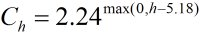This allows the extrapolation (again with a grain of salt) of approximate contour counts for larger sizes.

 Size Expected Contours h =  25 8,750,000 h =  30 493,000,000 h =  35 27,800,000,000 h =  40 1,570,000,000,000 h =  48 995,000,000,000,000

The total estimated count of all creature contours for sizes 1..48 is 1,800,000,000,000,000.

10.  Acknowledgements

Thanks to Professor Gilles Caporossi of HEC Montréal for initially suggesting a constructive approach that led to the enumeration method described above. Also thanks to Gunnar Brinkmann and Paul G. Mezey for related discussions on the enumeration of polyhex benzenoids.

Home - Games

Site designed by Cameron Browne © 2009.